Comparison Operators in SOQL : In our previous Salesforce tutorial we have learned about WHERE clause in SOQl. In this Salesforce Training Tutorial we are going to learn about Comparison operators in SOQL, Comparison operators with SOQL statement examples.

## Comparison Operators in SOQL

Comparison operators in SOQL are used to compare a value with another value to return TRUE or FALSE. Different types of comparison operators in SOQL(Salesforce Object Query Language) are

 Operator Description = Equals != Not Equals < Less than <= Less than or equal to > Greater than >= Greater than or equal to LIKE Like

### Equals Operator in SOQL

let us see an example using Equals operator to implement SOQL statements. Using Equal Operator in SOQL can retrieve the data based on criteria and condition equals “=”.

• SELECT Firstname, Lastname FROM Contact WHERE firstname = ‘Adarsh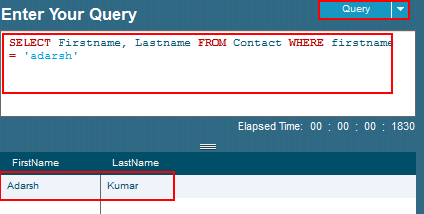From above SOQL statement the equals ” = ” checks whether the values of the two operands are equal. If the value is equal, the condition becomes ” TRUE ” . If not the condition Fails.

### Not Equals Operator.

Let us see an example using Not equals operator to implement SOQL Statements. Using Not Equals ” != “ data can retrieved based on the condition or Criteria.

• SELECT firstname, lastname FROM Contact WHERE firstname != ‘adarsh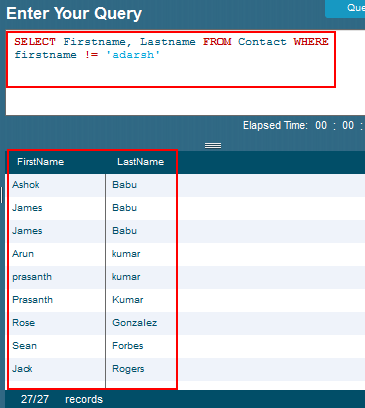From the above SOQL statement the date is retrieved from all the contacts whose first name not equals to Adarsh.

### Less than or Equal to Operator in SOQL

Let us see an example on Less than or Equal to operator in SOQL. using this operator we can retrieve records that are less than or equal to the given limit.

• SELECT name, Fee_paid__c FROM student__c WHERE fee_paid__c  <= 4000.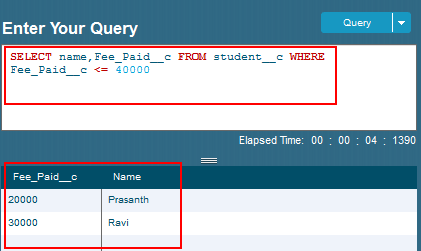Less than or equal to operator is used to check whether the values of the left operand is less than or equal to the value of the right operand. If the condition satisfies the condition will be TRUE.

From above SOQL statement the students who paid fee less than or equals to 40000 will be filtered.

### Greater than or Equal to operator in SOQL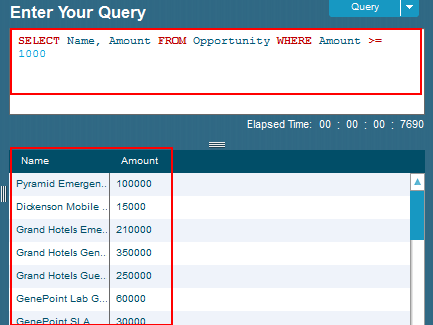### Greater than Operator in SOQL.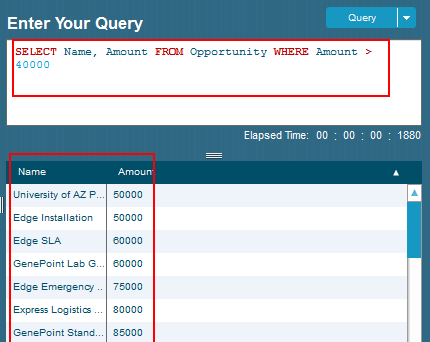### LIKE operator in SOQL

Like operator is used to retrieve the data from the matched substring provided. LIKE operator is also called as pattern matching filtering technique. We have to use ”  % ” character to include names ending with “express